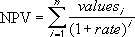Everyday Calculation

Free calculators and unit converters for general and everyday use.

Calculators » Financial » Net present value

NPV Calculator

Our online tools will provide quick answers to your calculation and conversion needs. On this page, you can calculate net present value (NPV) of an investment based on a series of cash flows that occur at regular time intervals. This NPV calculator works for annual, semi-annual, quarterly, monthly, weekly and daily cash flows.

% p.a.

Cash flow

× ( )

Result window

Android: Use this NPV calculator offline with our TVM financial calculator app.

NPV calculation

We use the same formula as implemented in MS-Excel for calculating net present value. The formula of NPV is:where, n is the number of cash flows

The discount rate rate is the periodic rate. In this calculator, you enter the discount rate as annual percentage rate; it will be automatically converted to it's equivalent periodic rate depending on the frequency of payment.

For explanation of NPV function, refer this Excel document.